# Third Chapter Lesson-13: Universality of NAND & NOR gates.

## At the end of this lesson-

• 1. You will be able to prove the universality of NAND and NOR gates.
• 2. You will be able to implement AND, OR & NOT gate using only NAND gate.
• 3. You will be able to implement AND, OR & NOT gate using only NOR gate.
• 4. You will be able to implement XOR & XNOR gate using only NAND gate.
• 5. You will be able to implement XOR & XNOR gate using only NOR gate.

## Proof of Universality of NAND Gate:

Implementation of NOT gate using only NAND gate: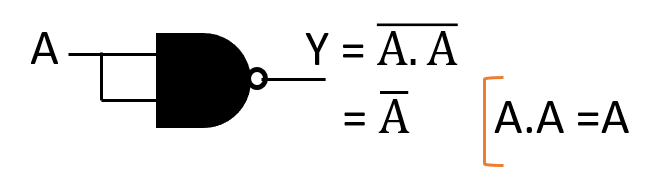Implementation of AND gate using only NAND gate: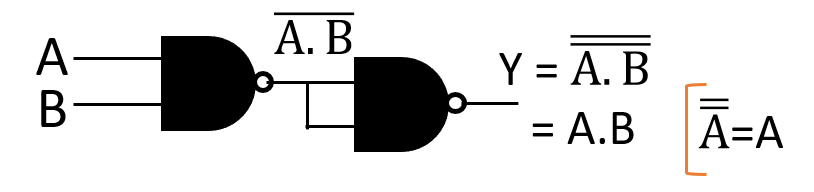Implementation of OR gate using only NAND gate:As we can see from the above discussion, All three basic gates can be implemented by only NAND Gate. Again we know that any gate or any circuit can be implemented by three basic gates. Since NAND Gate can implement any gate or any circuit with three basic gates (AND, OR, NOT), NAND Gate is called a universal Gate.

## Proof of Universality of NOR Gate:

Implementation of NOT gate using only NOR gate:Implementation of OR gate using only NOR gate:Implementation of AND gate using only NOR gate: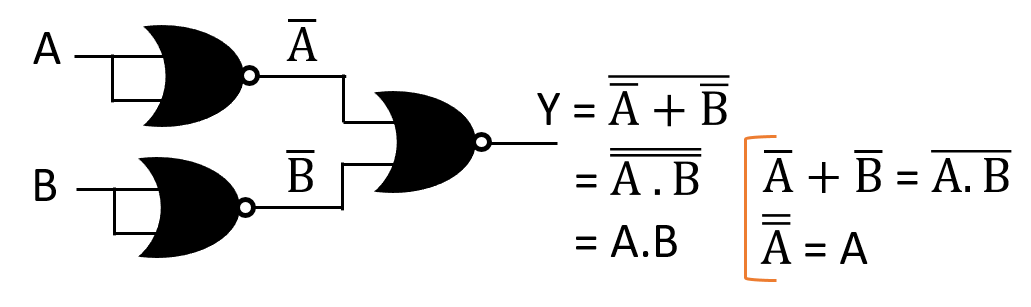As we can see from the above discussion, All three basic gates can be implemented by only NOR Gate. Again we know that any gate or any circuit can be implemented by three basic gates. Since NOR Gate can implement any gate or any circuit with three basic gates (AND, OR, NOT), NOR Gate is called a universal Gate.

[When implementing any function using only NAND gate, you should see if the function has any OR operator? If so, convert the OR to AND via the De Morgan’s theorem and then implement.]

## Implementation of 2-input XOR gate using only NAND gate:

Boolean function of the XOR gate for variables A and B,## Implementation of 2-input XNOR gate using only NAND gate:

Boolean function of the XNOR gate for variables A and B,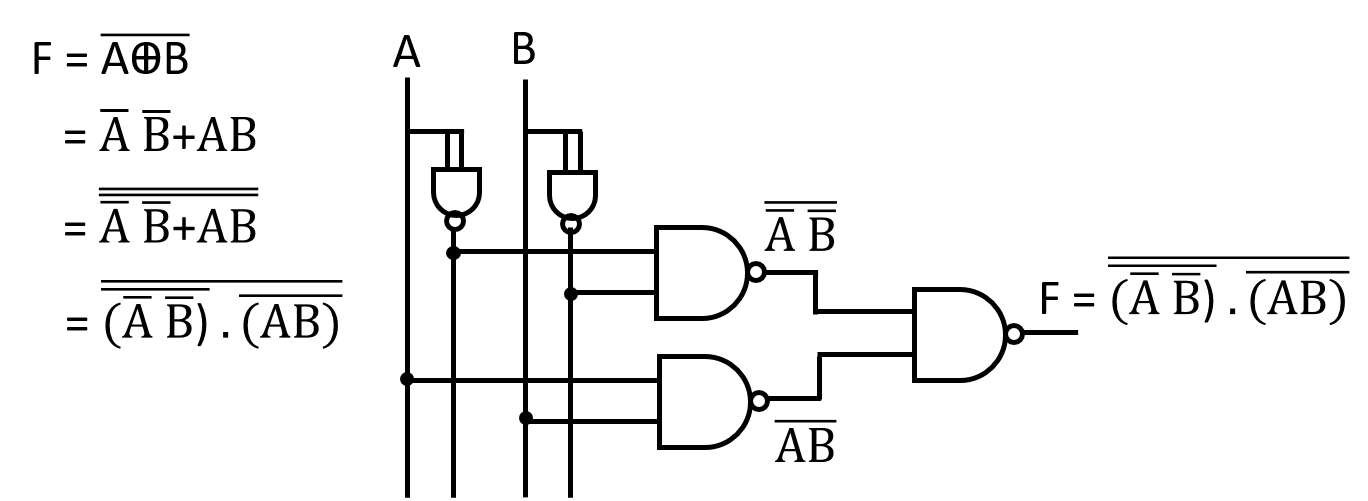[When implementing any function using only NOR gate, you should see if the function has any AND operator? If so, convert the AND to OR via the De Morgan’s theorem and then implement.]

## Implementation of 2-input XOR gate using only NOR gate:

Boolean function of the XOR gate for variables A and B,

## Implementation of 2-input XNOR gate using only NOR gate:

Boolean function of the XNOR gate for variables A and B,## Implement the following functions using only NAND/NOR gate: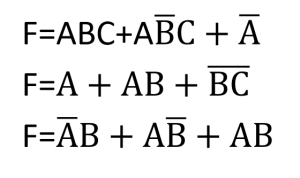## Lesson Evaluation-

Knowledge Based Questions:

Comprehension Based Questions:

• b. ‘NOR gate functions as a basic gate when all its inputs are same’ -explain.
• b. ‘NAND gate functions as a basic gate when all its inputs are same’ -explain.
• b. ‘XOR gate can be implemented using only NAND gate’ -explain.
• b. ‘XNOR gate can be implemented using only NAND gate’ -explain.
• b. ‘XOR gate can be implemented using only NOR gate’ -explain.
• b. ‘XNOR gate can be implemented using only NOR gate’ -explain.

Creative Questions:

According to the stem answer the following questions: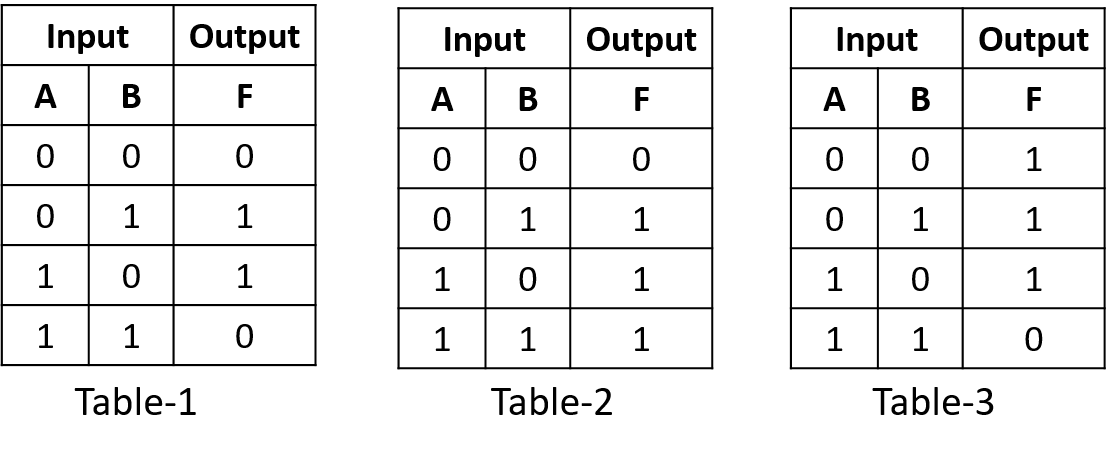c) How many gates of Table-3 are needed to implement Table-2? Explain.

d) Is it possible to implement gates of truth table-1 using the gate of truth table-3? Analyze.

According to the stem answer the following questions:c) Implement fig-2 using fig-1.

d) Is it possible to implement fig-3 using only fig-2? Analyze.

According to the stem answer the following questions: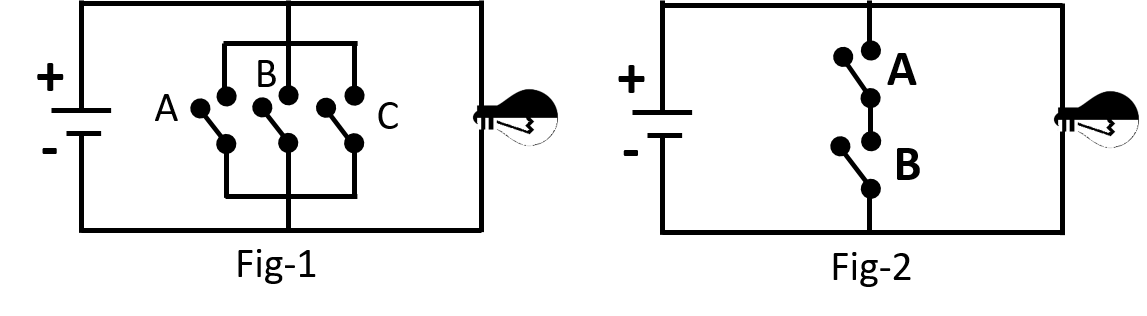c) Implement the basic gates using only the gate of Fig-2.

d) ‘It is possible to implement fig-1 using fig-2’ -Analyze.

Multiple Choice Questions:

According to the following stem answer the question no. 1 and 2: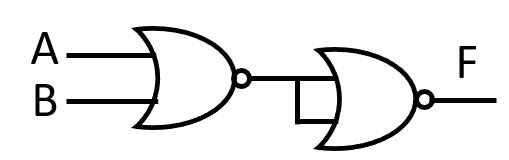1. Output of the circuit will be-

a) F= A+B      b) F= AB      c) F= (A+B)´ d) F= (AB)´

2. Output of the circuit will be 1, when–

i. A=0, B=0       ii. A=1, B=0       iii. A=1, B=1

Which one is correct?

a) i ও ii     b) i ও iii     c) ii ও iii     d) i, ii ও iii

Written by,